--- title: "STATS 220" subtitle: "R Markdown`r emo::ji('knit')`" type: "lecture" date: "" output: xaringan::moon_reader: css: ["assets/remark.css"] lib_dir: libs nature: ratio: 16:9 highlightStyle: github highlightLines: true countIncrementalSlides: false --- class: middle ```{r initial, echo = FALSE, cache = FALSE, results = 'hide'} library(knitr) options(htmltools.dir.version = FALSE, htmltools.preserve.raw = FALSE, tibble.width = 60, tibble.print_min = 6) opts_chunk\$set( echo = TRUE, warning = FALSE, message = FALSE, comment = "#>", fig.path = 'figure/', cache.path = 'cache/', cache = TRUE, fig.retina = 3, fig.align = 'center', fig.width = 4.5, fig.height = 4, fig.show = 'hold', dpi = 120 ) ``` ```{r xaringan-panelset, echo = FALSE} xaringanExtra::use_panelset() ``` .pull-left[ .center[ [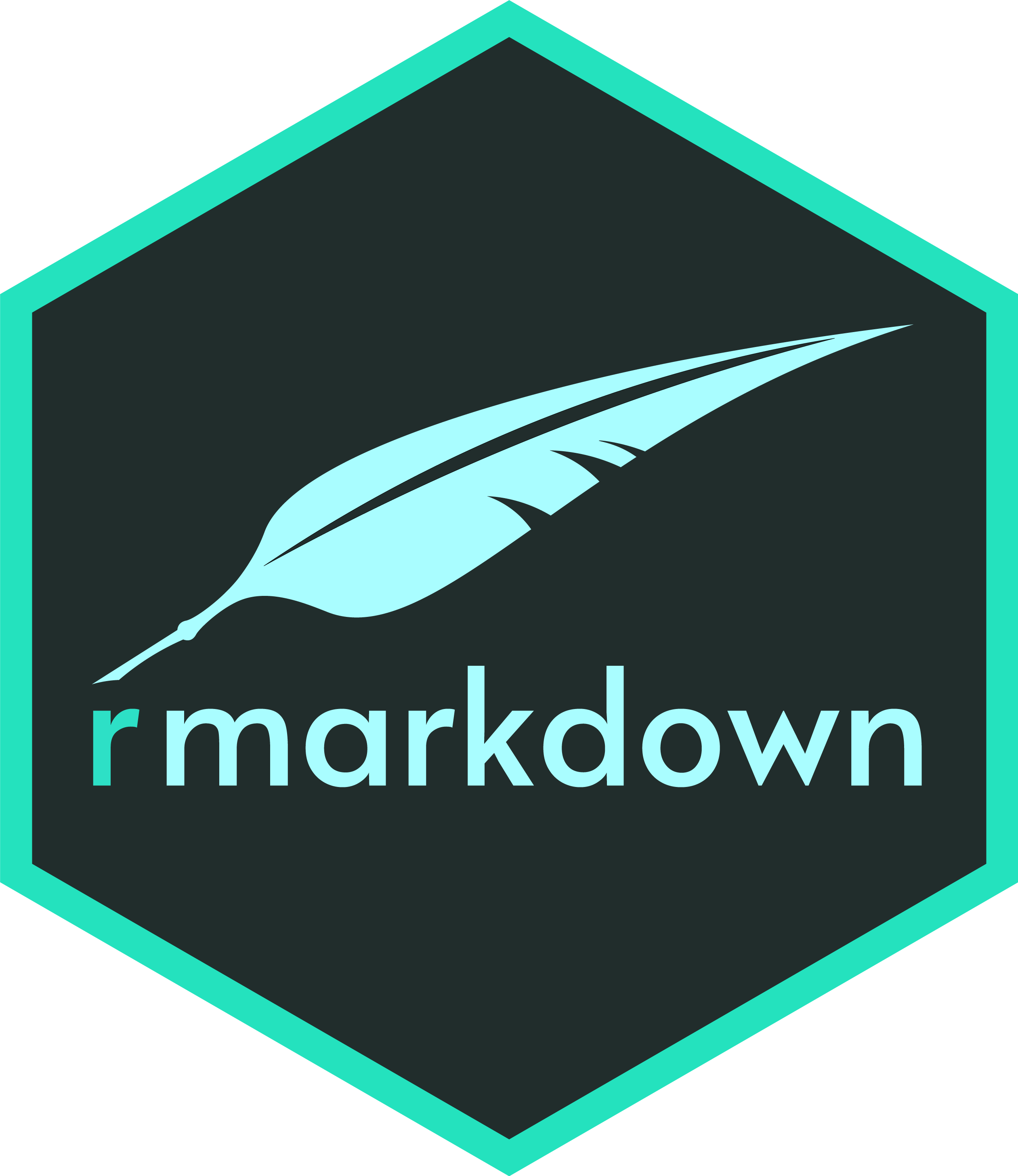](https://rmarkdown.rstudio.com) [](https://bookdown.org/yihui/blogdown/) [](http://bookdown.org) [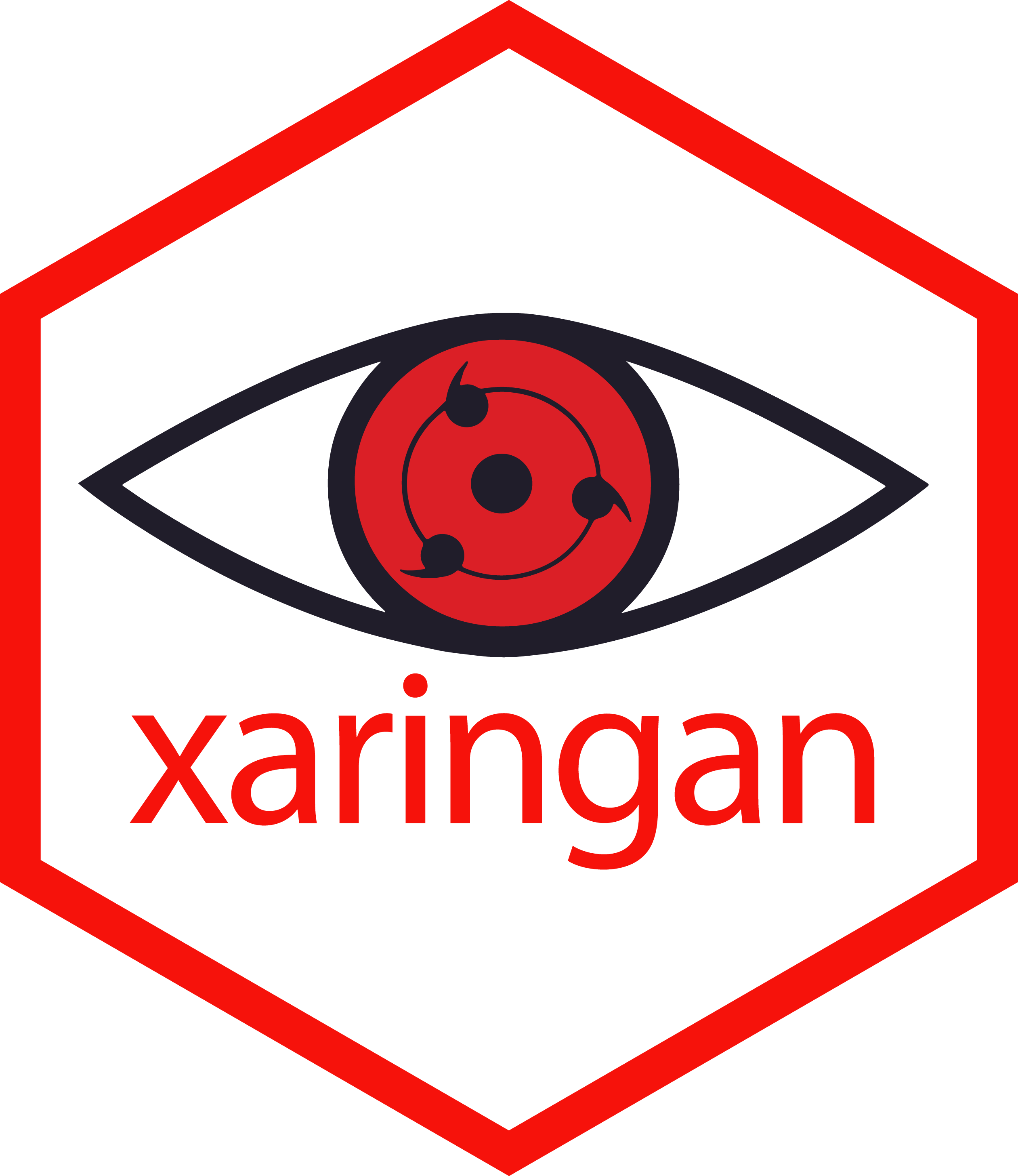](http://slides.yihui.org/xaringan/) ] ] .pull-right[ ## .center[Dynamic documents] * Combine code, rendered output (such as figures), and prose * Reproduce your analyses * Collaborate and share code with others * Communicate your results with others ] ??? * graphics for communication * keep learning communication * Rmd: a tool for integrating prose, code, and results * one md file for multiple documents --- class: inverse middle center # .large[.large[.large[]]] + .large[.large[.large[.blue[]]]] ??? Rmd: glue 2 things together --- ## Markdown .blue[] `.md` * Created by [John Gruber](https://daringfireball.net/projects/markdown/) & [Aaron Swartz](http://www.aaronsw.com) in 2004 * A lightweight .brown[markup language] to add formatting elements to plain-text documents, contrasting to WYSIWYG * Portable, platform independent, everywhere (e.g. Piazza, but not Canvas `r emo::ji("angry")`)??? * md originated back to 2004, but emerged around 2010 * aaron, reddit, a loss * The course website: write in md, and render in html by hugo * commonmark: it's one of markdown flavours, richer features. e.g. github * learned it in 5 mins, unlike R --- class: center middle ## .blue[] Basic syntaxMore on [Markdown syntax](https://www.markdownguide.org/basic-syntax/) ```{r md-syntax, echo = FALSE, eval = FALSE} library(gt) tibble::tribble( ~ md, ~ rendered, "`## Heading level 2`", "## Heading level 2", "`**bold**`", "**bold**", "`*italic*`", "*italic*", "\\`inline code\\`", "`inline code`", "`* apple`\n\n`* orange`", "* apple\n * orange", "`1. first`\n\n`2. second`", "1. first\n 2. second", "``", "", "`[stats220](http://stats220.earo.me)`", "[stats220](http://stats220.earo.me)" ) %>% gt() %>% fmt_markdown(everything()) %>% cols_label( md = md("**Markdown**"), rendered = md("**Rendered Output**") ) as_raw_html() ``` --- class: inverse background-image: url(https://raw.githubusercontent.com/allisonhorst/stats-illustrations/master/rstats-blanks/rmarkdown_wizards_blank.png) background-size: 100% 100% --- .pull-left[ ## Create R Markdown `.Rmd`
.center[] ] .pull-right[ ````markdown --- title: "STATS220 R Markdown demo" author: "Earo Wang" date: "``r 'r'` lubridate::today()`" output: html_document --- ```{r setup, include = FALSE}`r ''` library(knitr) opts_knit\$set(root.dir = here::here()) opts_chunk\$set(echo = TRUE) ``` ## R Markdown You can embed an R code chunk like this: ```{r gapminder, message = FALSE}`r ''` library(tidyverse) gp <- read_rds("data/gapminder.rds") gp ``` See . ```` ] --- .pull-left[ ## YAML header YAML is commonly used for configuration files, and starts and ends with `---` ````markdown ``r 'r'` lubridate::today()` ```` `r emo::ji("arrow_up")` inline executable R code * one markdown file, many formats + `pdf_document` + `xaringan::moon_reader` .small[ To compile to PDF, ```r install.packages('tinytex') tinytex::install_tinytex() ``` ] ] .pull-right[ ````markdown *--- *title: "STATS220 R Markdown demo" *author: "Earo Wang" *date: "``r 'r'` lubridate::today()`" *output: html_document *--- ```{r setup, include = FALSE}`r ''` library(knitr) opts_knit\$set(root.dir = here::here()) opts_chunk\$set(echo = TRUE) ``` ## R Markdown You can embed an R code chunk like this: ```{r gapminder, message = FALSE}`r ''` library(tidyverse) gp <- read_rds("data/gapminder.rds") gp ``` See . ```` ] --- .pull-left[ ## R chunk for global settings ````markdown ```{r setup, include = FALSE}`r ''` library(knitr) opts_knit\$set(root.dir = here::here()) opts_chunk\$set(echo = TRUE) ``` ```` `r emo::ji("arrow_up")` executable R chunk * `r`: language engine * `setup`: unique chunk name * `include`: include chunk output or not * `echo`: display source code or not ] .pull-right[ ````markdown --- title: "STATS220 R Markdown demo" author: "Earo Wang" date: "``r 'r'` lubridate::today()`" output: html_document --- *```{r setup, include = FALSE}`r ''` *library(knitr) *opts_knit\$set(root.dir = here::here()) *opts_chunk\$set(echo = TRUE) *``` ## R Markdown You can embed an R code chunk like this: ```{r gapminder, message = FALSE}`r ''` library(tidyverse) gp <- read_rds("data/gapminder.rds") gp ``` See . ```` ] --- .pull-left[ ## Write in markdown
.center[Weave together narrative text and code]

.center[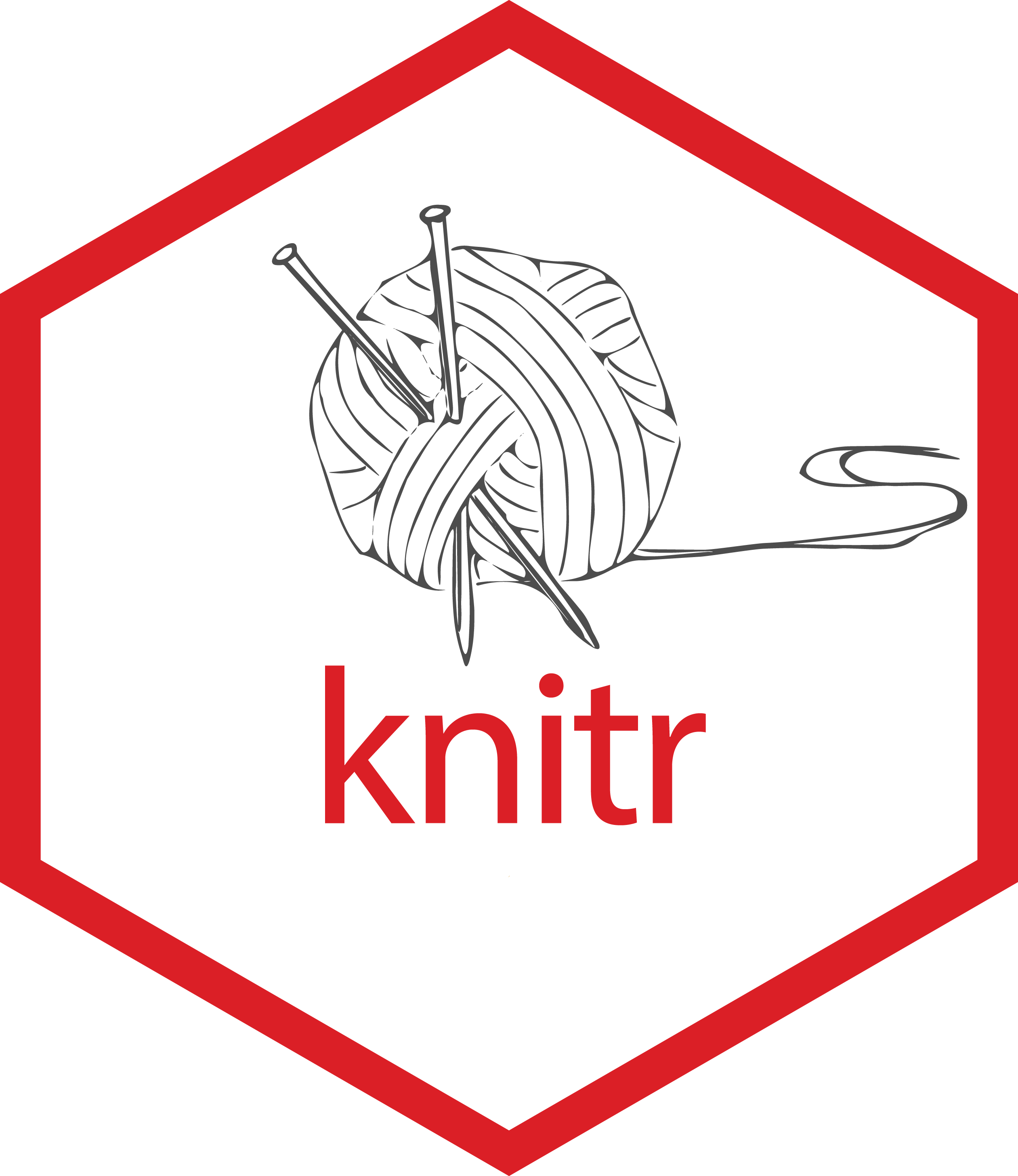] ] .pull-right[ ````markdown --- title: "STATS220 R Markdown demo" author: "Earo Wang" date: "``r 'r'` lubridate::today()`" output: html_document --- ```{r setup, include = FALSE}`r ''` library(knitr) opts_knit\$set(root.dir = here::here()) opts_chunk\$set(echo = TRUE) ``` *## R Markdown *You can embed an R code chunk like this: ```{r gapminder, message = FALSE}`r ''` library(tidyverse) gp <- read_rds("data/gapminder.rds") gp ``` *See . ```` ] --- .pull-left[ ## R chunk * `mpg`: unique chunk name * `message`: suppress message or not ```{r tidyverse, message = TRUE, cache = FALSE} library(tidyverse) ``` ] .pull-right[ ````markdown --- title: "STATS220 R Markdown demo" author: "Earo Wang" date: "``r 'r'` lubridate::today()`" output: html_document --- ```{r setup, include = FALSE}`r ''` library(knitr) opts_knit\$set(root.dir = here::here()) opts_chunk\$set(echo = TRUE) ``` ## R Markdown You can embed an R code chunk like this: *```{r gapminder, message = FALSE}`r ''` *library(tidyverse) *gp <- read_rds("data/gapminder.rds") *gp *``` See . ```` ] --- .pull-left[ ## Render

3 ways to render an `.Rmd` 1. click2. shortcut: Ctrl/Cmd + Shift + K 3. `rmarkdown::render("demo.Rmd")` ] .pull-right[] --- ````r ```{r scatterplot, fig.align = "center", fig.width = 4.5, fig.height = 4}`r ''` ggplot(gp, aes(gdpPercap, lifeExp)) + geom_point() + scale_x_log10() ``` ```` ```{r rmd-gapminder, message = FALSE, echo = FALSE} library(tidyverse) gp <- read_rds("data/gapminder.rds") ``` ```{r rmd-scatterplot, fig.align = "center", fig.width = 4.5, fig.height = 4, echo = FALSE} ggplot(gp, aes(gdpPercap, lifeExp)) + geom_point() + scale_x_log10() ``` --- ````r ```{r scatterplot, fig.align = "center", fig.width = 4.5, fig.height = 4}`r ''` ggplot(gp, aes(gdpPercap, lifeExp)) + geom_point() + scale_x_log10() ``` ```` .pull-left[ ## Chunk options * `scatterplot` gives the chunk a name. * `fig.align`: alignment of figures * `fig.width`/`fig.height` * [other figure options](https://yihui.org/knitr/options/#plots) **Good practice**: naming every single chunk! ] .pull-right[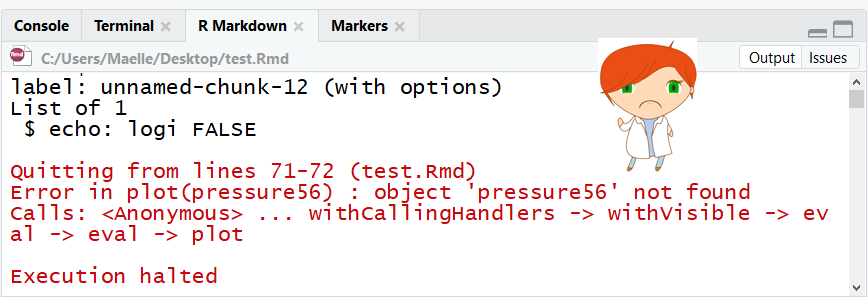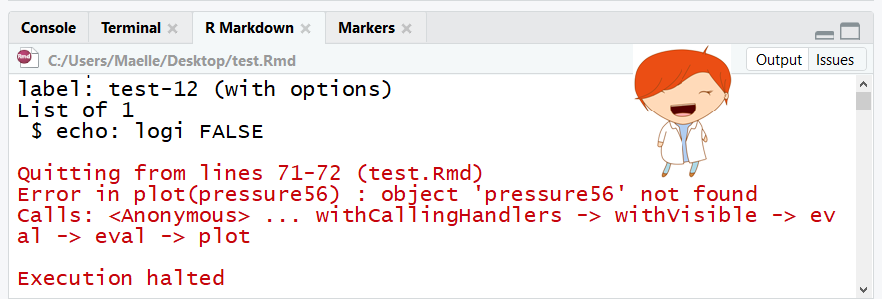.footnote[image credit: Maelle Salmon] ] --- ````r ```{r show-code, ref.label = "scatterplot", eval = FALSE}`r ''` ``` ```` .pull-left[ ## Reuse chunks by reference * `ref.label`: labels of the chunks from which the code of the current chunk is inherited * `eval`: evaluate the code chunk **Good practice**: naming every single chunk! ] .pull-right[ ```{r right-plot, ref.label = "rmd-scatterplot", echo = FALSE, fig.height = 3.5, fig.width = 4} ``` ] --- ```{css, echo = FALSE} table { margin: auto; border-top: 1px solid #666; border-bottom: 1px solid #666; font-size: 22px; } table thead th { border-bottom: 1px solid #ddd; } th, td { padding: 5px; } thead, tfoot, tr:nth-child(even) { background: #eee; } ``` ````r ```{r lm, echo = FALSE, cache = TRUE}`r ''` fit <- lm(lifeExp ~ log10(gdpPercap), data = gp) kable(coef(summary(fit))) ``` ```` .pull-left[ ## Chunk options * `echo`: overwrites the global setting * `cache`: evaluate once and skip for the future `kable()` generates a very simple HTML table. `r emo::ji("arrow_right")` ] .pull-right[ ```{r gapminder, echo = FALSE} gp <- read_rds("data/gapminder.rds") ```

```{r lm, echo = FALSE} fit <- lm(lifeExp ~ log10(gdpPercap), data = gp) kable(coef(summary(fit))) ``` ] --- ## Beyond : language engines ````py *```{python hello}`r ''` # remotes::install_github("rstudio/reticulate") x = "hello, python world!" print(x.split(" ")) ``` ```` ```{python hello, echo = FALSE} x = "hello, python world!" print(x.split(" ")) ```
````sh *```{sh head}`r ''` head -4 data/pisa/pisa-student.csv ``` ```` ```{sh pisa-header, echo = FALSE} head -4 data/pisa/pisa-student.csv ``` --- ## Beyond : language engines ````r ```{r db}`r ''` library(RSQLite) db <- dbConnect(SQLite(), dbname = "data/pisa/pisa-student.db") ``` *```{sql query, connection = db, output.var = "pisa18"}`r ''` SELECT * FROM pisa WHERE year = 2018 ``` ```` ```{r db, echo = FALSE} library(RSQLite) db <- dbConnect(SQLite(), dbname = "data/pisa/pisa-student.db") ``` ```{sql sql, connection = db, echo = FALSE, output.var = "pisa18"} SELECT * FROM pisa WHERE year = 2018 ``` ```{r r-pisa, echo = FALSE} tibble::as_tibble(pisa18) ``` --- ## Beyond : language engines ```{r eng} names(knitr::knit_engines\$get()) ``` --- ## Reading .pull-left[ .center[[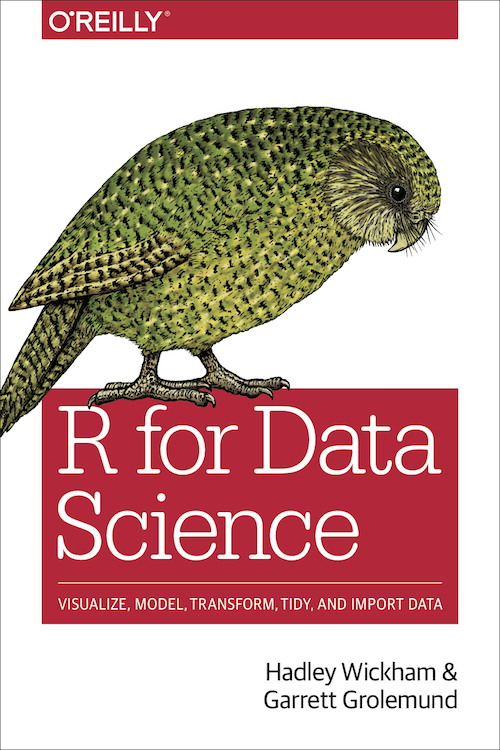](https://r4ds.had.co.nz)] ] .pull-right[ * [R Markdown](https://r4ds.had.co.nz/r-markdown.html) * [R Markdown formats](https://r4ds.had.co.nz/r-markdown-formats.html) * [R Markdown cheat sheet](https://www.rstudio.com/wp-content/uploads/2016/03/rmarkdown-cheatsheet-2.0.pdf?_ga=2.253787336.159731575.1620189500-118347454.1620189500) * [R Markdown reference guide](https://www.rstudio.com/wp-content/uploads/2015/03/rmarkdown-reference.pdf?_ga=2.253787336.159731575.1620189500-118347454.1620189500) ]